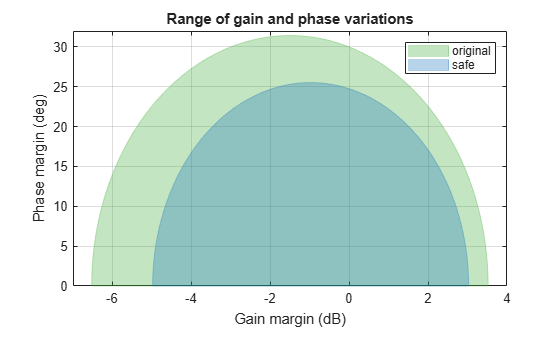# uscale

Scale uncertainty of block or system

## Syntax

``blk_scaled = uscale(blk,factor)``
``M_scaled = uscale(M,factor)``

## Description

example

````blk_scaled = uscale(blk,factor)` scales the amount of uncertainty in an uncertain control design block by `factor`. Typically, `factor` is a robustness margin returned by `robstab` or `robgain`, or a robust performance returned by `musynperf`. The uncertain element `blk_scaled` is of the same type as `blk`, with the amount of uncertainty scaled in normalized units. For instance, if `factor` is 0.75, the normalized uncertainty of `blk_scaled` is 75% of the normalized uncertainty of `blk`.```

example

````M_scaled = uscale(M,factor)` scales all the uncertain blocks in the model `M`. Non-uncertain elements are not changed. ```

## Examples

collapse all

Consider a feedback loop with the following open-loop gain.

`L = tf(3.5,[1 2 3 0]);`

Suppose that the system has gain uncertainty of 1.5 (gain can increase or decrease by a factor of 1.5) and phase uncertainty of ±30°.

```DGM = getDGM(1.5,30,'tight'); F = umargin('F',DGM)```
```F = Uncertain gain/phase "F" with relative gain change in [0.472,1.5] and phase change of ±30 degrees. ```

Examine the robust stability of the closed-loop system.

```T = feedback(L*F,1); SM = robstab(T)```
```SM = struct with fields: LowerBound: 0.8303 UpperBound: 0.8319 CriticalFrequency: 1.4482 ```

`robstab` shows that the system can only tolerate 0.83 times the modeled uncertainty before going unstable. Scale the `umargin` block `F` by this amount to find the largest gain and phase variation that the system can tolerate.

```factor = SM.LowerBound; Fsafe = uscale(F,factor)```
```Fsafe = Uncertain gain/phase "F" with relative gain change in [0.563,1.42] and phase change of ±24.8 degrees. ```

The scaled uncertainty has smaller ranges of both gain variation and phase variation. Compare these ranges for the original modeled variation and the maximum tolerable variation.

```DGM = F.GainChange; DGMsafe = Fsafe.GainChange; diskmarginplot([DGM;DGMsafe]) legend('original','safe')```Consider the uncertain control system of the example "Robust Performance of Closed-Loop System" on the `robgain` reference page. That example examines the sensitivity of the closed-loop response at the plant output to disturbances at the plant input.

```k = ureal('k',10,'Percent',40); delta = ultidyn('delta',[1 1]); G = tf(18,[1 1.8 k]) * (1 + 0.5*delta); C = pid(2.3,3,0.38,0.001); S = feedback(1,G*C)```
```S = Uncertain continuous-time state-space model with 1 outputs, 1 inputs, 4 states. The model uncertainty consists of the following blocks: delta: Uncertain 1x1 LTI, peak gain = 1, 1 occurrences k: Uncertain real, nominal = 10, variability = [-40,40]%, 1 occurrences Type "S.NominalValue" to see the nominal value, "get(S)" to see all properties, and "S.Uncertainty" to interact with the uncertain elements. ```

Suppose that you do not want the peak gain of this sensitivity function to exceed 1.5. Use `robgain` to find out how much of the modeled uncertainty the system can tolerate while the peak gain remains below 1.5.

`perfmarg = robgain(S,1.5)`
```perfmarg = struct with fields: LowerBound: 0.7821 UpperBound: 0.7837 CriticalFrequency: 7.8565 ```

With that performance requirement, the system can only tolerate about 78% of the modeled uncertainty. Scale all the uncertain elements in `S` to create a model of the closed-loop system with the maximum level of uncertainty that meets the performance requirement.

```factor = perfmarg.LowerBound; S_scaled = uscale(S,factor)```
```S_scaled = Uncertain continuous-time state-space model with 1 outputs, 1 inputs, 4 states. The model uncertainty consists of the following blocks: delta: Uncertain 1x1 LTI, peak gain = 0.782, 1 occurrences k: Uncertain real, nominal = 10, variability = [-31.3,31.3]%, 1 occurrences Type "S_scaled.NominalValue" to see the nominal value, "get(S_scaled)" to see all properties, and "S_scaled.Uncertainty" to interact with the uncertain elements. ```

The display shows how the uncertain elements in `S_scaled` have changed: the peak gain of the `ultidyn` element `delta` is reduced from 1 to 0.78, and the range of variation of the uncertain real parameter `k` is reduced from ±40% to ±31.3%.

## Input Arguments

collapse all

Uncertain control design block to scale, specified as a `ureal`, `umargin`, `ultidyn`, or other uncertain block.

Scaling factor, specified as a scalar. This argument is the amount by which `uscale` scales the normalized uncertainty of `blk` or `M`. For instance, if `factor` = 0.8, then the function reduces the uncertainty to 80% of its original value, in normalized units. Similarly, if `factor` = 2, then the function doubles the uncertainty.

Typically, `factor` is a robustness margin returned by `robstab` or `robgain`, or a robust performance returned by `musynperf`. Thus, you can use `uscale` to find the largest range of modeled uncertainty in a system for which the system has good robust stability or performance.

Uncertain model, specified as a `uss`, `umat`, `ufrd`, or `genss` with uncertain control design blocks. The `uscale` command scales uncertain control design blocks in `M`. Other blocks of `M` are unchanged.

## Output Arguments

collapse all

Scaled uncertain block, returned as a block of the same type as `blk`, such as a `ureal`, `umargin`, `ultidyn`, or other uncertain block. The uncertainty of `blk_scaled` is the same as the uncertainty in `M`, scaled by `factor`.

Scaled uncertain model, returned as a model of the same type as `M`, such as a `uss`, `umat`, `ufrd`, or `genss` with uncertain control design blocks. The uncertain control design blocks in `M_scaled` are the same as the blocks in `M`, with the size of uncertainty scaled by `factor` in normalized units.